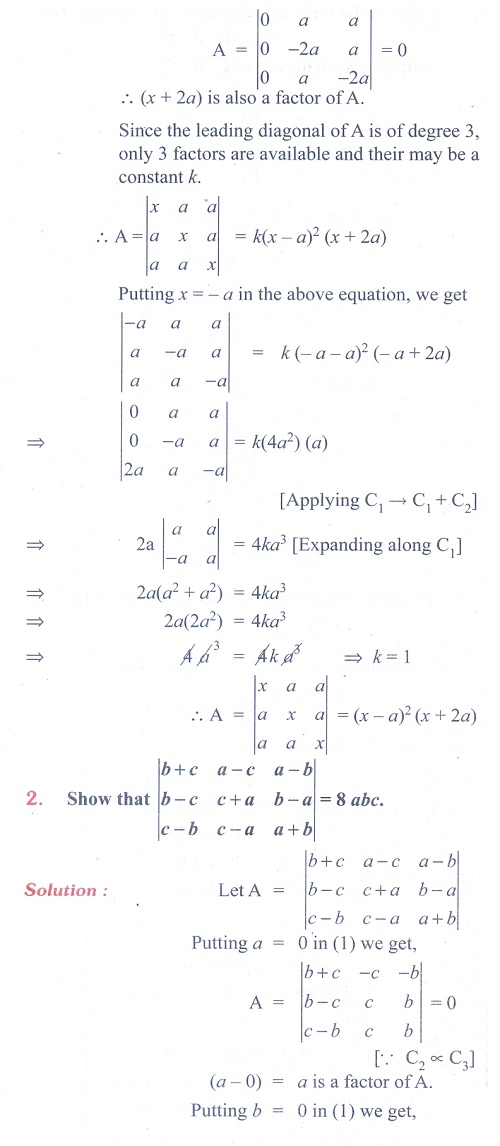Home | | Maths 11th std | Exercise 7.3: Application of Factor Theorem to Determinants

# Exercise 7.3: Application of Factor Theorem to Determinants

Maths Book back answers and solution for Exercise questions - Mathematics : Determinants: Application of Factor Theorem to DeterminantsTags : Problem Questions with Answer, Solution | Mathematics , 11th Mathematics : UNIT 7 : Matrices and Determinants
Study Material, Lecturing Notes, Assignment, Reference, Wiki description explanation, brief detail
11th Mathematics : UNIT 7 : Matrices and Determinants : Exercise 7.3: Application of Factor Theorem to Determinants | Problem Questions with Answer, Solution | Mathematics